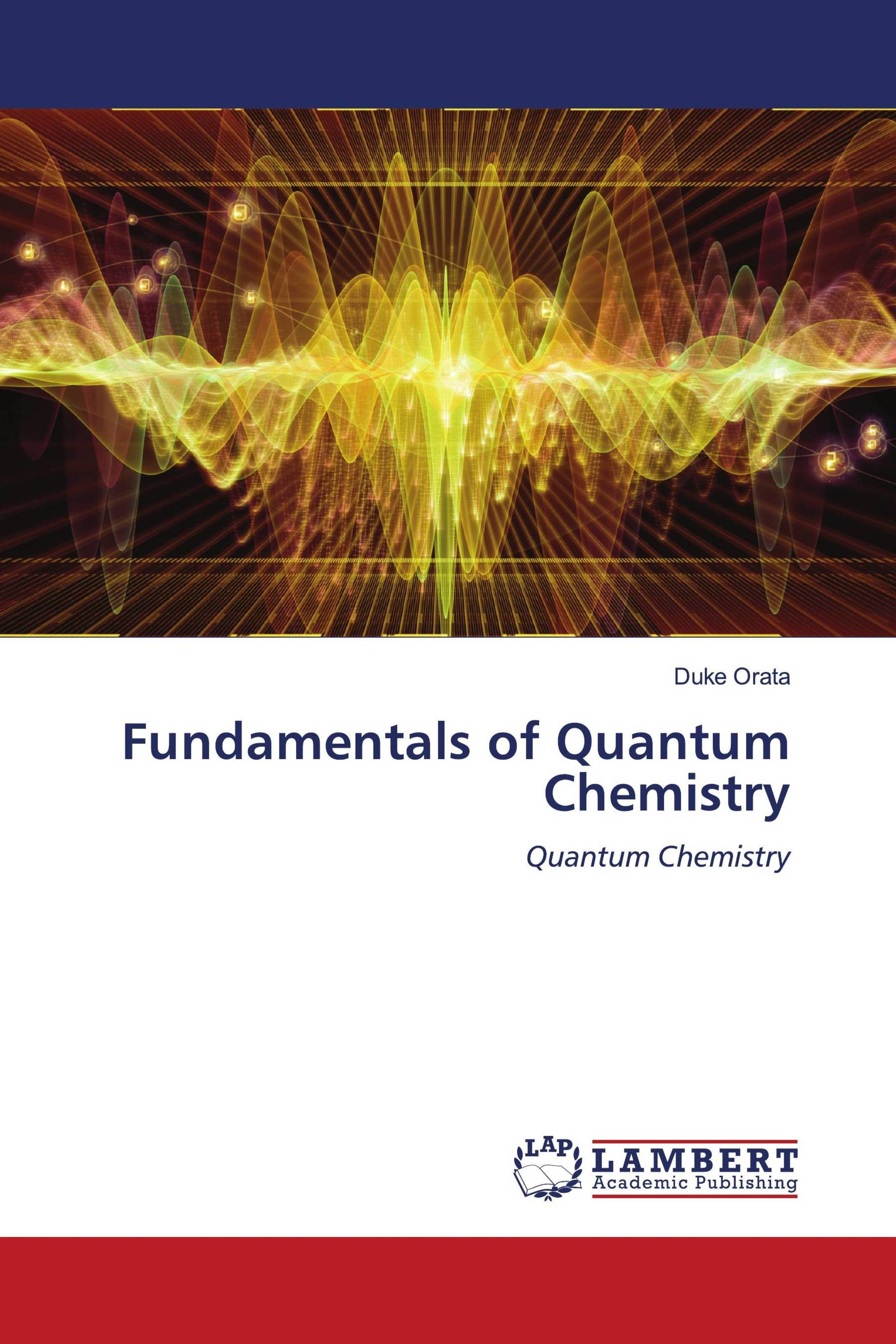• library@msu.ac.th
• Academic Resource Center Mahasarakham University
• library@msu.ac.th
• Academic Resource Center Mahasarakham Universityหมวดหมู่### Fundamentals Of Quantum Chemistry

Fundamentals of Quantum Chemistry discusses in a simplified manner the fundamental quantum chemistry principles and processes and show in a step wise manner the derivation of key equations in Quantum Chemistry. Concepts discussed in this textbook include elements of classical mechanics, wave particle duality, the wave function, eigenvalue equations, application of quantum theory ,harmonic motion, pertubation theory, electron spin, the variation method and self-consistent field method in Quantum chemistry.

ใส่ตะกร้า
• ISBN9786204735726
• ประเภท E-Book
• ผู้แต่ง Duke Orata
• สำนักพิมพ์ LAP LAMBERT Academic Publishing
• ครั้งที่พิมพ์ 1
• ปีที่พิมพ์2022
• ภาษาภาษาอังกฤษ
• หมวดหมู่วิทยาศาสตร์
###### : ข้อมูลหนังสือ

Fundamentals of Quantum Chemistry discusses in a simplified manner the fundamental quantum chemistry principles and processes and show in a step wise manner the derivation of key equations in Quantum Chemistry. Concepts discussed in this textbook include elements of classical mechanics, wave particle duality, the wave function, eigenvalue equations, application of quantum theory ,harmonic motion, pertubation theory, electron spin, the variation method and self-consistent field method in Quantum chemistry.